×#### Thank you for registering.

One of our academic counsellors will contact you within 1 working day.

Click to Chat

1800-1023-196

+91-120-4616500

CART 0

• 0

MY CART (5)

Use Coupon: CART20 and get 20% off on all online Study Material

ITEM
DETAILS
MRP
DISCOUNT
FINAL PRICE
Total Price: Rs.

There are no items in this cart.
Continue Shopping• Complete JEE Main/Advanced Course and Test Series
• OFFERED PRICE: Rs. 15,900
• View Details

```Chapter 16: Surface Area And Volume Exercise – 16.2

Question: 1

Consider a tent cylindrical in shape and surmounted by a conical top having height 16 m and radius as common for all the surfaces constituting the whole portion of the tent which is equal to 24 m. Height of the cylindrical portion of the tent is 11 m. Find the area of Canvas required for the tent.

Solution:

The diameter of the cylinder given in the question is 24 m.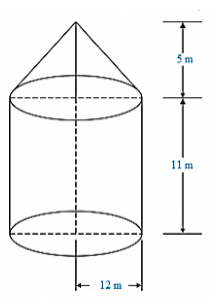Radius (R) = 12/2 = 12 m

The height of the Cylindrical part (H1) given in the question is 11m

So, Height of the cone part (H2) = 5 m

Now,

Vertex of the cone above the ground = 11 + 5 = 16 m

Curved Surface area of the Cone (say, S1) = πRL = 22/7 × 6 × L

Where,So,

Curved Surface Area of Cone (S1) = 22/7 × 12 × 13               .... E.1

Curved Surface Area of Cylinder (S2) = 2πRH1

S2 = 2π(12)(11) m2              .... E.2

To find the area of Canvas required for tent

S = S1 + S2 = E.1 + E.2

S = 22/7 × 12 × 13 + 2 × 22/7 × 12 × 11

S = 490 + 829.38

S = 1319.8 m2

S = 1320 m2

Hence, the total Canvas required for tent (s) = 1320 m2

Question: 2

Consider a Rocket. Suppose the rocket is in the form of a Circular Cylinder Closed at the lower end with a Cone of the same radius attached to its top. The Cylindrical portion of the rocket has radius say, 2.5 m and the height of that cylindrical portion of the rocket is 21m. The Conical portion of the rocket has a slant height of 8m, then calculate the total surface area of the rocket and also find the volume of the rocket.

Solution:

Given radius of the cylindrical portion of the rocket (say, R) = 2.5 m

Given height of the cylindrical portion of the rocket (say, H) = 21 m

Given Slant Height of the Conical surface of the rocket (say, L) = 8 m

Curved Surface Area of the Cone (say S1) = RL

S1 = m2            .... E.1

Curved Surface Area of the Cone (say, S2) = 2RH + R2

S2 = (2π × 2.5 × 21) + π (2.5)2

S2 = (π × 10.5) + (π ×6.25)       ... E.2

So, The total curved surface area = E.1 + E.2

S = S1 + S2

S = (π20) + (π105) + (π6.25)

S = 62.83 + 329.86 + 19.63

S = 412.3 m2

Hence, the total Curved Surface Area of the Conical Surface = 412.3 m2

Volume of the conical surface of the rocket = 1/3 × 22/7 × R2 × h

V1 = 1/3 × 22/7 × (2.5)2 × h      .... E.3

Let, h be the height of the conical portion in the rocket.

Now,

L2 = R2 + h2

h2 = L2 - R2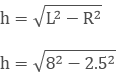h = 23.685 m

Putting the value of h in E.3, we will get

Volume of the conical portion (V1) = 1/3 × 22/7 × 2.52 × 23.685 m2              .... E.4

Volume of the Cylindrical Portion (V2) = πR2h

V2 = 22/7 × 2.52 × 21

So, the total volume of the rocket = V1 + V2

V = 461.84 m2

Hence, the total volume of the Rocket (V) is 461.84 m2

Question: 3

Take a tent structure in vision being cylindrical in shape with height 77 dm and is being surmounted by a cone at the top having height 44 dm. The diameter of the cylinder is 36 m. Find the curved surface area of the tent.

Solution:

As per the question,

Height of the tent = 77 dm

Height of a surmounted cone = 44 dm

Height of the Cylindrical Portion = Height of the tent – Height of the surmounted Cone

= 77 - 44

= 33 dm = 3.3 m

Given diameter of the cylinder (d) = 36 m

So, Radius (r) of the cylinder = 36/2

R = 18 m

Consider L as the Slant height of the Cone.

L2 = r2 + h2

L2 = 182 + 3.32

L2 = 324 + 10.89

L2 = 334.89

L = 18.3 m

Hence, Slant height of the cone (L) = 18.3 m

The Curved Surface area of the Cylinder (S1) = 2πRh

S1 = 2π184.4 m2  …… E.1

The Curved Surface area of the cone (S2) = πRh

S2 = π × 18 × 18.3 m2 …. E.2

So, the total curved surface of the tent = S1 + S2

S = S1 + S2

S = (2π18 × 4.4) + (π18 × 18.3)

S = 1532. 46 m2

Hence, the total Curved Surface Area (S) = 1532.46 m2

Question: 4

A toy is in the form of a cone surmounted on a hemisphere. The diameter of the base and the height of the cone are 6 cm and 4 cm, respectively. Determine the surface area of the toy.

Solution:

Given that,The height of the cone (h) = 4 cm

Diameter of the cone (d) = 6 cm

So, radius (r) = 3 [as we know that the radius is half of the diameter]

Let, 'l' be the slant height of cone. Then,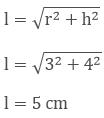So, slant height of the cone (l) = 5 cm

Curved surface area of the cone (S1) = πrl

S1 = π(3)(5)

S1 = 47.1 cm2

Curved surface area of the hemisphere (S2) = 2πr2

S2 = 2π(3)2

S2 = 56.23 cm2

So, the total surface area (S) = S1 + S2

S = 47.1 + 56.23

S = 103.62 cm2

Therefore, the curved surface area of the toy = S = 103.62 cm2

Question: 5

A solid is in the form of a right circular cylinder, with a hemisphere at one end and a cone at the other end. The radius of the common base is 3.5 cm and the height of the cylindrical and conical portions are 10 cm and 6 cm, respectively. Find the total surface area of the solid. (Use π = 22/7).

Solution:

Given that,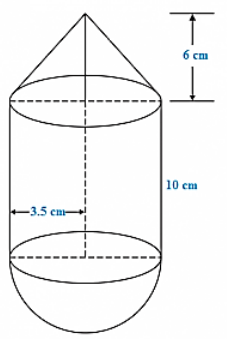Radius of the common base (r) = 3.5 cm

Height of the cylindrical part (h) = 10 cm

Height of the conical part (H) = 6 cm

Let, 'l' be the slant height of the cone, then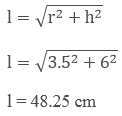Curved surface area of the cone (S1) = πrl

S1 = π(3.5)(48.25)

S1 = 76.408 cm2

Curved surface area of the hemisphere (S2) = 2πrh

S2 = 2π(3.5) (10)

S2 = 220 cm2

So, the total surface area (S) = S1 + S2

S = 76.408 + 220

S = 373.408 cm2

Question: 6

A toy is in the shape of a right circular cylinder with a hemisphere on one end and a cone on the other. The radius and height of the cylindrical parts are 5cm and 13 cm, respectively. The radii of the hemispherical and conical parts are the same as that of the cylindrical part. Find the surface area of the tor if the total height of the toy is 30 cm.

Solution:

As per the parameters given in the question, we have

Height of the Cylindrical portion = 13 cm

Radius of the Cylindrical portion = 5 cm

Height of the whole solid = 30 cm

Then,

The curved surface area of the Cylinder (say S1) = 2πrh

S1 = 2π(5)(13)

S1 = 408.2 cm2

The curved surface area of the cone (say S2) = πrL

S2 = π(6)L

For conical part, we have

h = 30 - 13 - 5 = 12 cm

We know that,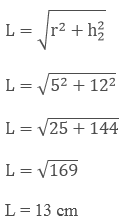So, The curved surface area of the cone (say S2) = πrl

S2 = π(5)(13) cm2

S2 = 204.1 cm2

The curved surface area of the hemisphere (say S3) = 2πr2

S3 = 2π(5)2

S3 = 157 cm2

The total curved surface area (say S) = S1 + S2 + S3

S = (408.2 + 204.1 + 157)

S = 769.3 cm2

Therefore, the surface area of the toy (S) = 769.3 cm2

Question: 7

Consider a cylindrical tub having radius as 5 cm and its length 9.8 cm. It is full of water. A solid in the form of a right circular cone mounted on a hemisphere is immersed in tub. If the radius of the hemisphere is 3.5 cm and the height of the cone outside the hemisphere is 5 cm. Find the volume of water left in the tub.

Solution:

As per the parameters given in the question, we have

The radius of the Cylindrical tub (r) = 5 cm

Height of the Cylindrical tub (say H) = 9.8 cm

Height of the cone outside the hemisphere (say h) = 5 cm

Radius of the hemisphere = 5 cm

Now,

The volume of the Cylindrical tub (say V1) = πr2H

V1 = π(5)2 9.8

V1 = 770 cm3

The volume of the Hemisphere (say V2) = 2/3 × π × r3

V2 = 2/3 × 22/7 × 3.53

V2 = 89.79 cm3

The volume of the Hemisphere (say V3) = 23 × π × r × 2h

V3 = 2/3 × 22/7 × 3.52 × 5

V3 = 64.14 cm3

Therefore, The total volume = Volume of the cone + Volume of the hemisphere

= V2 + V3

V = 89.79 + 64.14 cm3

= 154 cm3

Hence, the total volume of the solid = 154 cm3

To find the volume of the water left in the tube, we have to subtract the volume of the hemisphere and the cone from the volume of the cylinder.

Hence, the volume of water left in the tube = V

= V1 - V2

V = 770 - 154

V = 616 cm3

Therefore, the volume of water left in the tube is 616 cm3.

Question: 8

A circus tent has a cylindrical shape surmounted by a conical roof. The radius of the cylindrical base is 20 cm. The height of the cylindrical and conical portions is 4.2 cm and 2.1 cm.  Find the volume of that circus tent.

Solution:

As per the parameters given in the question, we have

Radius of the cylindrical portion (say R) = 20 m

Height of the cylindrical portion (say h1) = 4.2 m

Height of the conical portion (say h2) = 2.1 m

Now,

Volume of the Cylindrical portion (say V1) = πr2 h1

V1 = π(20)24.2

V1 = 5280 m3

Volume of the conical part (say V2) = 1/3 × 22/7 × r2 × h2

V2 = 13 × 22/7 × 202 × 2.1

V2 = 880 m3

Therefore, the total volume of the tent (say V) = volume of the conical portion + volume of the Cylindrical portion

V = V1 + V2

V = 6160 m3

Volume of the tent = V = 6160 m3

Question: 9

A petrol tank is a cylinder of base diameter 21 cm and length 18 cm fitted with the conical ends, each of axis 9 cm. Determine the capacity of the tank.

Solution:

As per the parameters given in the question, we have

Base diameter of the Cylinder = 21 cm

Radius (say r) = diameter/2 = 25/2 = 11.5 cm

Height of the Cylindrical portion of the tank (say h1) = 18 cm

Height of the Conical portion of the tank (say h2) = 9 cm

Now,

The volume of the Cylindrical portion (say V1) = πr2 h1

V1 = π(11.5)2 18

V1 = 7474.77 cm3

The volume of the Conical portion (say V2) = 1/3 × 22/7 × r2 × h2

V2 = 1/3 × 22/7 × 11.52 × 9

V2 = 1245.795 cm3

Therefore, the total volume of the tank (say V) = volume of the conical portion + volume of the Cylindrical portion

V = V1 + V2

V = 8316 cm3

So, the capacity of the tank = V = 8316 cm3

Question: 10

A conical hole is drilled in a circular cylinder of height 12 cm and base radius 5 cm. The height and base radius of the cone are also the same. Find the whole surface and volume of the remaining Cylinder.

Solution:

As per the parameters given in the question, we haveHeight of the circular Cylinder (say h1) = 12 cm

Base radius of the circular Cylinder (say r) = 5 cm

Height of the conical hole = Height of the circular cylinder, i.e., h1 = h2 = 12 cm

Base radius of the conical hole = Base radius of the circular Cylinder = 5 cm

Let us consider, L as the slant height of the conical hole.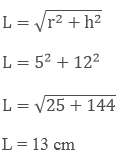Now,

The total surface area of the remaining portion in the circular cylinder (say V1) = πr2 + 2πrh + πrl

V1 = π(5)2  + 2π(5)(12) + π(5)(13)

V1 = 210 π cm2

Volume of the remaining portion of the circular cylinder = Volume of the cylinder – Volume of the conical hole

V = πr2h - 1/3 × 22/7 × r2 × h

V = π(5)2(12) - 1/3 × 22/7 × 52 × 12

V = 200 π cm2

Therefore, the volume of the remaining portion of the cylindrical part = V = 200 π cm2

Question: 11

A tent is in the form of a cylinder of diameter 20 m and height 2.5 m surmounted by a cone of equal base and height 7.5 m. Find the capacity of tent and the cost of canvas as well at a price of Rs.100 per square meter.

Solution:

As per the parameters given in the question, we have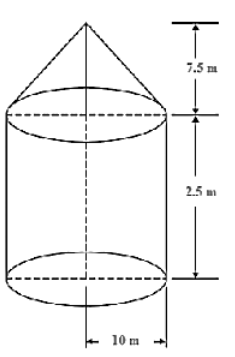Diameter of the cylinder = 20 m

Radius of the cylinder = 10 m

Height of the cylinder (say h1) = 2.5 m

Radius of the cone = Radius of the cylinder (say r) = 15 m

Height of the Cone (say h2) = 7.5 m

Let us consider L as the slant height of the Cone, then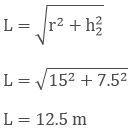Volume of the cylinder = πr2h1 = V1

V1 = π(10)2 2.5

V1 = 250 πm3

Volume of the Cone = 1/3 × 22/7 × r2 × h2 = V2

V2 = 1/3 × 22/7 × 102 × 7.5

V2 = 250π m3

Therefore, The total capacity of the tent = volume of the cylinder + volume of the cone = V1 + V2

V = 250 π + 250 π

V = 500π m3

Hence, the total capacity of the tent = V = 4478.5714 m3

The total area of the canvas required for the tent is S = 2 πrh1 + πrl

S = 2(π)(10)(2.5) + π(10)(12.5)

S = 550 m2

Therefore, the total cost of the canvas is (100) (550) = Rs. 55000

Question: 12

Consider a boiler which is in the form of a cylinder having length 2 m and there’s a hemispherical ends each of having a diameter of 2 m. Find the volume of the boiler.

Solution:

As per the parameters given in the question, we have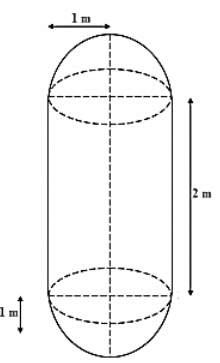Diameter of the hemisphere = 2 m

Radius of the hemisphere (say r) = 1 m

Height of the cylinder (say h1) = 2 m

The volume of the Cylinder = πr2h1 = V1

V1 = π(1)22

V1 = 22/7 × 2 = 44/7m3

Since, at each of the ends of the cylinder, hemispheres are attached.

So,

The volume of two hemispheres = 2 × 2/3 × 22/7 × r3 = V2

V2 = 2 × 2/3 × 22/7 × 13

V2 = 22/7 × 4/3 = 88/21 m3

Therefore, the volume of the boiler = volume of the cylindrical portion + volume of the two hemispheres = V

V = V1 + V2

V = 44/7 + 88/21

V = 220/21 m3

So, The volume of the boiler = V = 220/21 m3

Question: 13

A vessel is a hollow cylinder fitted with a hemispherical bottom of the same base. The depth of the cylinder is 14/3 and the diameter of the hemisphere is 3.5 m. Calculate the volume and the internal surface area of the solid.

Solution:

As per the parameters given in the question, we have

Diameter of the hemisphere = 3.5 m

Radius of the hemisphere (say r) = 1.75 m

Height of the cylinder (say h) = 14/3 m

The volume of the Cylinder = πr2 h1 = V1

V1 = π(1.75)2143 m3

The volume of two hemispheres = 2 × 2/3 × 22/7 × r3 = V2

V2 = 2 × 2/3 × 22/7 × 1.753 m3

Therefore, The total volume of the vessel = volume of the cylinder + volume of the two hemispheres = V

V = V1 + V2

V = 56 m3

Therefore, Volume of the vessel = V = 56 m3

Internal surface area of solid (S) = 2 πr h1 + 2 πr2

S = Surface area of the cylinder + Surface area of the hemisphere

S = 2 π(1.75)(143) + 2 π(1.75)2

S = 70.51 m3

Hence, the internal surface area of the solid = S = 70.51 m3

Question: 14

Consider a solid which is composed of a cylinder with hemispherical ends. If the complete length of the solid is 104 cm and the radius of each of the hemispherical ends is 7 cm. Find the cost of polishing its surface at the rate of Rs. 10 per dm2.

Solution:

As per the parameters given in the question, we have

Radius of the hemispherical end (say r) = 7 cm

Height of the solid = (h + 2r) = 104 cm

The curved surface area of the cylinder (say S) = 2 πr h

S = 2 π(7) h ... E.1

⇒ h + 2r = 104

⇒ h = 104 − (2 × 7)

h = 90 cm

Put the value of h in E.1, we will get

S = 2 π(7)(90)

S = 3948.40 cm2

So, the curved surface area of the cylinder = S = 3948.40 cm2

Curved surface area of the two hemisphere (say SA) = 2 (2πr2)

SA = 22π(7)2

SA = 615.75 cm2

Therefore, the total curved surface area of the solid = Curved surface area of the cylinder + Curved surface area of the two hemisphere = TSA

TSA = S + SA

TSA = 3948.40 + 615.75

TSA = 4571.8cm2 = 45.718 dm2

The cost of polishing the 1 dm2 surface of the solid is Rs. 15

So, the cost of polishing the 45.718 dm2 surface of the solid = 10 45.718 = Rs. 457.18

Hence,

The cost of polishing the whole surface of the solid is Rs. 457.18.

Question: 15

A cylindrical vessel of diameter 14 cm and height 42 cm is fixed symmetrically inside a similar vessel of diameter 16cm and height of 42 cm. The total space between the two vessels is filled with Cork dust for heat insulation purposes. Find how many cubic cms of the Cork dust will be required?

Solution:

As per the parameters given in the question, we have

Depth of the cylindrical vessel = Height of the cylindrical vessel = h = 42 cm

Inner diameter of the cylindrical vessel = 14 cm

Inner radius of the cylindrical vessel = r1 = 14/2 = 7 cm (as we know that the radius is half of the diameter)

Outer diameter of the cylindrical vessel = 16 cm

Outer radius of the cylindrical vessel = r2 = 16/2 = 8 cm (as we know that the radius is half of the diameter)

Now,

The volume of the cylindrical vesselV = 1980 cm3

Therefore, Volume of the vessel = V = 1980 cm3 = Amount of cork dust required.

Question: 16

A cylindrical road roller made of iron is 1 m long. Its internal diameter is 54 cm and the thickness of the iron sheet used in making roller is 9 cm. Find the mass of the road roller if 1 cm3 of the iron has 7.8 gm mass.

Solution:

As per the parameters given in the question, we have]

Height of the cylindrical road roller = h = 1 m = 100 cm

Internal Diameter of the cylindrical road roller = 54 cm

Internal radius of the cylindrical road roller = 27 cm = r (as we know that the radius is half of the diameter)

Given the thickness of the road roller (T) = 9 cm

Let us assume that the outer radii of the cylindrical road roller be R.

T = R - r

9 = R - 27

R = 27 + 9

R = 36 cm

Now,

The volume of the iron sheet = π × (R2 − r2) × h = V

V = π × (362 − 272) × 100

V = 1780.38 cm3

So, the volume of the iron sheet = V = 1780.38 cm3

Mass of 1 cm3 of the iron sheet = 7.8 gm

So, the mass of 1780.38 cm3 of the iron sheet = 1388696.4gm = 1388.7 kg

Hence, the mass of the road roller (m) = 1388.7 kg

Question: 17

A vessel in the form of a hollow hemisphere mounted by a hollow cylinder. The diameter of the hemisphere is 14 cm and the total height of the vessel is 13cm. Find the inner surface area of the vessel.

Solution:

As per the parameters given in the question itself, we have

Diameter of the hemisphere = 14 cm

Radius of the hemisphere = 7 cm (as we know that the radius is half of the diameter)

Total height of the vessel = 13 cm = h + r

Now,

Inner surface area of the vessel = 2r (h + r) = SA

SA = 2 (13) (7)

SA = 182 cm2 = 572 cm2

Therefore, the inner surface area of the vessel = SA = 572 cm2

Question: 18

A toy is in the form of a cone of radius 3.5 cm mounted on a hemisphere of same radius. The total height of the toy is 15.5 cm. Find the total surface area of the toy.

Solution:

As per the parameters given in the question, we have

Radius of the conical portion of the toy = 3.5 cm = r

Total height of the toy = 15.5 cm = h

Length of the cone = L = 15.5 – 3.5 = 12 cm

Now,

The curved surface area of the cone = πrL = SA

SA = π(3.5)(12)

SA = 131.94 cm2

The curved surface area of the hemisphere = 2πr2 = S

S = 2π(3.5)2

S = 76.96 cm2

Therefore, The total surface area of the toy = Curved surface area of the cone + curved surface area of the hemisphere = TSA

TSA = 131.94 + 76.96

TSA = 208.90 cm2

Hence, the total surface area of the children’s toy = TSA = 209 cm2

Question: 19

The difference between outside and inside surface areas of the cylindrical metallic pipe 14 cm long is 44 dm2. If pipe is made of 99 cm2 of metal. Find outer and inner radii of the pipe.

Solution:

Let, inner radius of the pipe be r1.

Radius of outer cylinder be r2.

Length of the cylinder (h) = 14 cm

Difference between the outer and the inner surface area is 44 dm2.

So,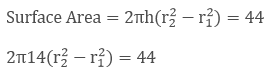(r2 − r1) = 1/2            .... E.1

So,

Volume of the metal used is 99 cm2,Therefore,

(r2 + r1) = 9/2                .... E.2

Solve E.1 and E.2 to get,

r2 = 5/2 cm

r1 = 2 cm

Question: 20

A right circular cylinder having diameter 12 cm and height 15 cm is full ice-cream. The ice-cream is to be filled in cones of height 12 cm and diameter 6 cm having a hemispherical shape on the top. Find the number of such cones which can be filled with ice-cream.

Solution:

Given,

Radius of cylinder (r1) = 6 cm

Radius of hemisphere (r2) = 3 cm

Height of cylinder (h) = 15 cm

Height of the cones (l) = 12 cm

Volume of cylinder = πr21h

= π × 62 ×15              .... E.1

Volume of each cone = Volume of cone + Volume of hemisphereLet, number of cones be 'n'

n(Volume of each cone) = Volume of cylinder

n(1/3 π3212 + 2/3 π33) = π(6)215

n = 50/5 = 10

So, the number of cones being filled with the cylinder = n = 10

Question: 21

Consider a solid iron pole having cylindrical portion 110 cm high and the base diameter of 12 cm is surmounted by a cone of 9 cm height. Find the mass of the pole. Assume that the mass of 1 cm3 of iron pole is 8 gm.

Solution:

As per the data given in the question, we have

Base diameter of the cylinder = 12 cm

Radius of the cylinder = 6 cm = r (as we know that the radius as half of the diameter)

Height of the cylinder = 110 cm = h

Length of the cone = 9 cm = L

Now,

The volume of the cylinder = π × r2 × h = V1

V1 = π × 62 × 110 cm3  ... E-1

The volume of the cone = V2 = 1/3 × πr2L

V2 = 1/3 × π6212

V2 = 108π cm3

Volume of the pole = volume of the cylinder + volume of the cone = V1 + V2 = V

V = 108π + π(6)2 110

V = 12785.14 cm3

Given mass of 1 cm3 of the iron pole = 8 gm

Then, mass of 12785.14 cm3 of the iron pole = 8

12785.14 = 102281.12 gm = 102.2 kg

Therefore, the mass of the iron pole = 102.2 kg

Question: 22

A solid toy is in the form of a hemisphere surmounted by a right circular cone. Height of the cone is 2 cm and the diameter of the base is 4 cm. If a right circular cylinder circumscribes the toy, find how much more space it will cover?

Solution:

Given that,

Radius of the cone, cylinder and hemisphere (r) = 2 cm

Height of the cone (l) = 2 cm

Height of the cylinder (h) = 4 cm

Volume of the cylinder = π × r2 × h = V1

V1 = π × 22 × 4 cm3         .... E-1

Volume of the cone = V2 = 1/3 π r2l

V2 = 1/3 × π × 22 × 2

V2 = 1/3 × π4 × 2 cm3               .... E - 2

Volume of the hemisphere = V3= 2/3 π r32

V3 = 2/3 × π × 23 cm3

V3 = 2/3 π × 8 cm3          .... E - 3

So, remaining volume of the cylinder when the toy is inserted to it = V1 - (V2 + V3)

V = 16π - 8π = 8π cm3

Hence, remaining volume of the cylinder when toy is inserted into it = V = 8π cm3

Question: 23

Consider a solid consisting of a right circular cone of height 120 cm and radius 60 cm standing on a hemisphere of radius 60 cm, is placed upright in the right circular cylinder full of water such that it touches bottoms. Find the volume of the water left in the cylinder, if radius of the cylinder is 60 cm and its height is 180 cm.

Solution:

As per the data given in the question, we have

Radius of the circular cone = r = 60 cm

Height of the circular cone = L = 120 cm

Radius of the hemisphere = r = 60 cm

Radius of the cylinder = R = 60 cm

Height of the cylinder = H = 180 cm

Now,

Volume of the circular cone = V1 = 1/3 × πr2l

V1 = 1/3 × π × 602 × 120

V1 = 452571.429 cm3

Volume of the hemisphere = V2 = 2/3 × πr3

V2 = 2/3 × π × 603

V2 = 452571.429 cm3

Volume of the cylinder = π × R2 × H = V3

V3 = π × 602 × 180

V3 = 2036571.43 cm3

Volume of water left in the cylinder = Volume of the cylinder - (Volume of the circular cone + Volume of the hemisphere) = V

V = V3 - (V1 + V2)

V = 2036571.43 – (452571.429 + 452571.429)

V = 2036571.43 – 905142.858

V = 1131428.57 cm3

V = 1.1314 m3

Therefore, the volume of the water left in the cylinder = V = 1.1314 m3

Question: 24

Consider a cylindrical vessel with internal diameter 10 cm and height 10.5 cm is full of water. A solid cone of base diameter 8 cm and height 6 cm is completely immersed in water. Find the value of water when:

(i) Displaced out of the cylinder

(ii) Left in the cylinder

Solution:

As per the parameters given in the question, we have

Internal diameter of the cylindrical vessel = 10 cm

Radius of the cylindrical vessel = r = 5 cm (as we know that the radius is half of the diameter)

Height of the cylindrical vessel = h = 10.5 cm

Base diameter of the solid cone = 7 cm

Radius of the solid cone = R = 3.5 cm (as we know that the radius is half of the diameter)

Height of the cone = L = 6 cm

(i) Volume of water displaced out from the cylinder = Volume of the cone = V1

V1 = 1/3 × πR2L

V1 = 1/3 × π3.52 × 6

V1 = 77 cm3

Therefore, the volume of the water displaced after immersion of the solid cone in the cylinder = V1 =77 cm3

(ii) Volume of the cylindrical vessel = π × r2 × h = V2

V2 = π × 52 × 10.5

V2 = 824.6 cm3 = 825 cm3

Volume of the water left in the cylinder = Volume of the cylindrical vessel – Volume of the solid cone

V = V2 – V1

V = 825 - 77

V = 748 cm3

Therefore, the volume of the water left in cylinder = V = 748 cm3

Question: 25

A hemispherical depression is cut from one face of a cubical wooden block of the edge 21 cm such that the diameter of the hemispherical surface is equal to the edge of the cubical surface. Determine the volume and the total surface area of the remaining block.

Solution:

As per the data given in the question itself, we have

Edge of the cubical wooden block = e = 21 cm

Diameter of the hemisphere = Edge of the cubical wooden block = 21 cm

Radius of the hemisphere = 10.5 cm = r (as we know that the radius is half of the diameter)

Now,

Volume of the remaining block = Volume of the cubical block – Volume of the hemisphere

V = e3 − (2/3 πr3)

V = 213 − (2/3 π10.53)

V = 6835.5 cm3

Surface area of the block = 6(e2) = SA

SA = 6(212) ... E - 1

Curved surface area of the hemisphere = CSA = 2πr2

CSA = 2π10.52 ... E - 2

Base area of the hemisphere = BA = πr2

BA = π10.52 .... E - 3

So, Remaining surface area of the box = SA - (CSA + BA)

= 6(212) - (2π10.52 + π10.52)

= 2992.5 cm2

Therefore, the remaining surface area of the block = 2992.5 cm2

Volume of the remaining block = V = 6835.5 cm3

Question: 26

A boy is playing with a toy which is in the form of a hemisphere surmounted by a right circular cone of the same base radius as that of the hemisphere. If the radius of the base of the cone is 21 cm and its volume is 2/3 of the volume of the hemisphere. Calculate the height of the cone and surface area of the toy.

Solution:

As per the parameter given in the question itself, we have

Radius of the cone = 21 cm = R

Radius of the hemisphere = Radius of the cone = 21 cm

Volume of the cone = 2/3 of the hemisphere

We know that,

The volume of the cone = V1 = 1/3 × πR2L

V1 = 1/3 × π212L

Also, we know that,

The volume of the hemisphere = V2 = 2/3 × πR3

V2 = 2/3 × π213 cm3

Now, as per the condition

V1 = 2/3 V2

V1 = 2/3 ×169714.286

1/3 × π212L = 2/3×π213

L = 28 cm

Curved surface area of the Cone = CSA1 = πRL

CSA1 = π × 21 × 28 cm2

Curved Surface area of the hemisphere = CSA2 = 2πR2

CSA2 = 2π(212) cm2

Now, the total surface area = S = CSA1 + CSA2

S = π × 21 × 28 + 2π(212)

S = 5082 cm2

Therefore, the curved surface area of the toy = S = 5082 cm2

Question: 27

Consider a solid which is in the form of a cone surmounted on hemisphere. The radius of each of them is being 3.5 cm and the total height of the solid is 9.5 cm. Find the volume of the solid.

Solution:

As per the data given in the question, we have

Radius of the hemisphere = 3.5 cm = R

Radius of the cone = Radius of the hemisphere = 3.5 cm = R

Total height of the solid = 9.5 cm = H

Then,

Length of the cone = Total height – Radius of the cone

L = 9.5 – 3.5 = 6 cm

Now,

The volume of the cone = V1 = 1/3 × πR2L

V1 = 1/3 × π3.526 cm3  ... E-1

The volume of the hemisphere = V2 = 2/3 × πR3

V2 = 2/3 × π53 cm3  ... E-2

Total volume of the solid = Volume of the cone + Volume of the hemisphere = V

V = V1 + V2

V = 1/3 × π3.526 + 2/3 × π53

V = 166.75 cm3

So, the volume of the solid = V = 166.75 cm3

Question: 28

A wooden toy is made by scooping out a hemisphere of same radius from each end of the solid cylinder. If the height of the cylinder is 10 cm, and its base is of radius 3.5 cm, find the volume of the wood in the toy.

Solution:

Given that,

Radius of the cylinder = Radius of the hemisphere = 3.5 cm = r

Height of the hemisphere = 10 cm = h

Volume of the cylinder = π × r2 × h = V1

V1 = π × 3.52 × 10 .... E-1

Volume of the hemisphere = V2 = 2/3 × πr3

V2 = 2/3 × π3.53 cm3  .... E-2

So,

Volume of the wood in the toy = Volume of the cylinder - 2(Volume of the hemisphere)

V = V1 - V2

V = 205.33 cm3

Question: 29

The largest possible sphere is carved out of a wooden solid cube of side 7 cm. Find the volume of the wood left.

Solution:

Given that,

Diameter of the wooden solid = 7 cm

Radius of the wooden solid = 3.5 cm

Volume of the cube = a3

V1 = 3.53 …. E – 1.

Volume of sphere = 4/3 × π × r3 = V2

V2 = 4/3 × π × 3.53 .... E – 2

Volume of the wood left = Volume of the cube - Volume of sphere

V = 3.53 − 4/3 × π × 3.53

V = 163.33 cm3

Question: 30

From a solid cylinder of height 2.8 cm and diameter 4.2 cm, a conical cavity of the same height and same diameter is hollowed out. Find the total surface area of the remaining solid.

Solution:

Given that,

Height of the cylinder = 2.8 cm = Height of the cone

Diameter of the cylinder = 4.2 cm

Radius of the cylinder = 2.1 cm = Radius of the cone

CSA of the cylindrical part = CSA1 = 2πRH

CSA1 = 2π(2.8)(2.1) cm2

Curved surface area of the Cone = CSA2 = πRL

CSA2 = π × 2.1 × 2.8 cm2

Area of the cylindrical base = πr2 = π(2.1)2

Total surface area of the remaining solid = CSA of the cylindrical part + Curved surface area of the Cone + Area of the cylindrical base

TSA = 2π(2.8)(2.1) + π × 2.1 × 2.8 + π(2.1)2

TSA = 36.96 + 23.1 + 13.86

TSA = 73.92 cm2

Question: 31

The largest cone is carved out from one face of the solid cube of side 21 cm. Find the volume of the remaining solid.

Solution:

Given that,

The radius of the largest possible cone is carved out of a solid cube is equal to the half of the side of the cube.

Diameter of the cone = 21 cm

Radius of the cone = 10.5 cm

The height of the cone is equal to the side of the cone.

Volume of the cube = e3

V1 = 10.53 ....  E-1.

Volume of the cone = V2 = 1/3 × πr2L

V2 = 1/3 × π10.5221 cm3  ... E-2

Volume of the remaining solid = Volume of cube - Volume of cone

V = 10.53 - 1/3 × π10.5221

V = 6835.5 cm3

Question: 32

A solid wooden toy is in the form of a hemisphere surmounted by a cone of the same radius. The radius of the hemisphere is 3.5 cm and the total wood used in the making of toy is.Find the height of the toy. Also, find the cost of painting the hemispherical part of the toy at the rate of Rs. 10 per square cm.

Solution:

Given that,

Radius of the hemisphere = 3.5 cm

Volume of the solid wooden toyAs, Volume of the solid wooden toy = Volume of the cone + Volume of the hemisphere1/3 × πr2L + 2/3 × πr3 = 10016

1/3 × π3.52L + 2/3 × π3.53 = 10016

L + 7 = 13

L = 6 cm

Height of the solid wooden toy = Height of the cone + Radius of the hemisphere

= 6 + 3.5

= 9.5 cm

Now, curved surface area of the hemisphere = 2πR2

CSA2 = 2π(3.52) = 77 cm2

Cost of painting the hemispherical part of the toy = 10 77 = Rs. 770

Question: 33

How many spherical bullets can be made out of a solid cube of lead whose edge measures 55 cm and each of the bullet being 4 cm in diameter?

Solution:

Let, the total number of bullets be a.

Diameter of the bullet = 4 cm

Radius of the spherical bullet = 2 cm (as we know that the radius is half of the diameter)

Now,

Volume of a spherical bullet = 4/3 × π × r3 = V

V = 4/3 × π × 23

V = 4/3 × 22/7 × 23

V = 33.5238 cm3

Volume of 'a' number of the spherical bullets = V a

V1 = (33.5238 a) cm23

Volume of the solid cube = (55)3 = 166375 cm3

Volume of 'a' number of the spherical bullets = Volume of the solid cube

33.5238 a = 166375

A = 4962.892

Hence, total number of the spherical bullets = 4963

Question: 34

Consider a children’s toy which is in the form of a cone at the top having a radius of 5 cm mounted on a hemisphere which is the base of the toy having the same radius. The total height of the toy is 20 cm. Find the total surface area of the toy.

Solution:

As per the parameters given in the question, we have

Radius of the conical portion of the toy = 5 cm = r

Total height of the toy = 20 cm = h

Length of the cone = L = 20 – 5 = 15 cm

Now,

The curved surface area of the cone = πrL = SA

SA = π(5)(15)

SA = 235.7142 cm2

The curved surface area of the hemisphere = 2πr2 = S

S = 2π(5)2

S = 157.1428 cm2

Therefore, The total surface area of the toy = Curved surface area of the cone + curved surface area of the hemisphere = TSA

TSA = 235.7142 + 157.1428

TSA = 392.857 cm2

Hence, the total surface area of the children’s toy = TSA = 392.857 cm2

Question: 35

A boy is playing with a toy conical in shape and is surmounted with hemispherical surface. Consider a cylinder in with the toy is inserted. The diameter of cone is the same as that of the radius of cylinder and hemispherical portion of the toy which is 8 cm. The height of the cylinder is 6 cm and the height of the conical portion of the toy is 3 cm. Assume a condition in which the boy’s toy is inserted in the cylinder, then find the volume of the cylinder left vacant after insertion of the toy.

Solution:

As per the parameter given in the question, we have

Diameter of the cone = Diameter of the Cylinder = Diameter of the Hemisphere = 8 cm

Radius of the cone = Radius of the cylinder = Radius of the Hemisphere = 4 cm = r (as we know that the radius is half of the diameter)

Height of the conical portion = 3 cm = L

Height of the cylinder = 6 cm = H

Now,

Volume of the cylinder = π × r2 × H = V1

V1 = π × 42 × 6

V1 = 301.7142 cm3

Volume of the Conical portion of the toy = V2 = 1/3 × πr2L

V2 = 1/3 × π42 × 3

V2 = 50.2857 cm3

Volume of the hemispherical portion of the toy = V3 = 2/3 × πr3

V3 = 2/3 × π43

V3 = 134.0952 cm3

So, the remaining volume of the cylinder when the toy (conical portion + hemispherical portion) is inserted in it = Volume of cylinder – (volume of the conical portion + volume of the hemispherical portion)

V = V1 – (V2 + V3)

V = 301.7142 – (50.2857 + 134.0952)

V = 301.7142 – 184.3809

V = 117.3333 cm3

Therefore, the remaining portion of the cylinder after insertion of the toy in it = V = 117.3333 cm3

Question: 36

Consider a solid consisting of a right circular cone of height 120 cm and radius 60 cm standing on a hemisphere of radius 60 cm, is placed upright in the right circular cylinder full of water such that it touches bottoms. Find the volume of the water left in the cylinder, if radius of the cylinder is 60 cm and its height is 180 cm.

Solution:

As per the data given in the question, we have

Radius of the circular cone = r = 60 cm

Height of the circular cone = L = 120 cm

Radius of the hemisphere = r = 60 cm

Radius of the cylinder = R = 60 cm

Height of the cylinder = H = 180 cm

Now,

Volume of the circular cone = V1 = 1/3 × πr2L

V1 = 1/3 × π602 × 120

V1 = 452571.429 cm3

Volume of the hemisphere = V2 = 2/3 × πr3

V2 = 2/3 × π603

V2 = 452571.429 cm3

Volume of the cylinder = π × R2 × H = V3

V3 = π × 602 × 180

V3 = 2036571.43 cm3

Volume of water left in the cylinder = Volume of the cylinder  (volume of the circular cone + volume of the hemisphere) = V

V = V3 – (V1 + V2)

V = 2036571.43 – (452571.429 + 452571.429)

V = 2036571.43 – 905142.858

V = 1131428.57 cm3

V = 1.1314 m3

Therefore, the volume of the water left in the cylinder = V = 1.1314 m3

Question: 37

Consider a cylindrical vessel with internal diameter 20 cm and height 12 cm is full of water. A solid cone of base diameter 8 cm and height 7 cm is completely immersed in water. Find the value of water when

(i) Displaced out of the cylinder

(ii) Left in the cylinder

Solution:

As per the parameters given in the question, we have

Internal diameter of the cylindrical vessel = 20 cm

Radius of the cylindrical vessel = r = 10 cm (as we know that the radius is half of the diameter)

Height of the cylindrical vessel = h = 12 cm

Base diameter of the solid cone = 8 cm

Radius of the solid cone = R = 4 cm (as we know that the radius is half of the diameter)

Height of the cone = L = 7 cm

(i) Volume of water displaced out from the cylinder = Volume of the cone = V1

V1 = 1/3 × πR2L

V1 = 1/3 × π42 × 7

V1 = 117.3333 cm3

Therefore, the volume of the water displaced after immersion of the solid cone in the cylinder = V1=117.3333 cm3

(ii) Volume of the cylindrical vessel = π × r2 × h = V2

V2 = π × 102 × 12

V2 = 3771.4286 cm3

Volume of the water left in the cylinder = Volume of the cylindrical vessel - Volume of the solid cone

V = V2 - V1

V = 3771.4286 - 117.3333

V = 3654.0953 cm3

Therefore, the volume of the water left in cylinder = V = 3654.0953 cm3
```### Course Features

• 728 Video Lectures
• Revision Notes
• Previous Year Papers
• Mind Map
• Study Planner
• NCERT Solutions
• Discussion Forum
• Test paper with Video Solution